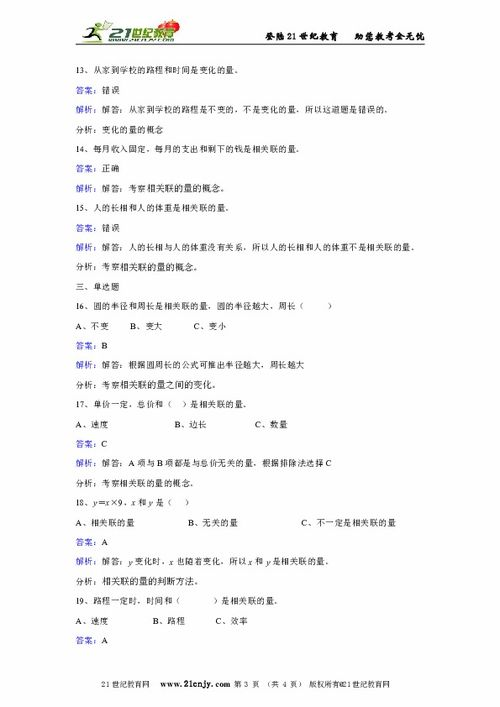# 四级下册数学第一节作业答案(四年级口算题带答案100道)

## 1.四年级口算题带答案100道

15*3= 15*30= 150*3= 150*30= 15*300= 3*150=25*4= 45*20= 50*40= 250*4= 125*4= 125*8=21*4= 21*40= 210*4= 60*90= 600*8= 60*50=43*2= 20*34= 3*230= 12*40= 70*20= 3*900=60*90= 12*7= 12*70= 120*70= 800*90= 40*200=35*20= 25*20= 500*30= 18*5= 25*4= 350*20=14*5= 140*50= 28*30= 30*20= 40*40= 140*20=11*60= 13*30= 130*30= 50*40= 40*200= 50*600=30*310= 70*8= 410*20= 20*10= 16*100= 200*70=50*200= 32*30= 13*300= 40*800= 121*4= 60*900=10*460= 120*3= 20*500= 70*20= 250*4= 120*50=15*30= 25*30= 250*3= 150*40= 25*300= 30*150=25*40= 45*200= 70*40= 150*4= 125*8= 125*4=210*4= 200*40= 310*3= 80*90= 600*7= 600*50=43*20= 20*35= 3*210= 120*60= 700*20= 30*240=600*90= 12*8= 120*60= 500*70= 80*100= 90*200=350*20= 450*20= 500*20= 16*50= 45*4= 350*10=140*5= 14*30= 26*30= 60*200= 340*20= 180*50=110*60= 11*300= 230*30= 550*4= 440*20= 50*800=20*320= 700*8= 450*20= 200*10= 17*100= 500*70=60*200= 34*30= 19*300= 80*800= 122*4= 70*900=10*100= 12*300= 50*500= 700*60= 125*8= 160*50=50*200= 32*30= 13*300= 40*800= 121*4= 60*900=100*46= 220*3= 60*500= 700*20= 150*4= 220*40=25*30= 250*30= 250*3= 450*20= 75*20= 30*150=45*200= 15*600= 700*40= 150*200= 25*8= 25*40=310*20= 200*50= 110*9= 800*30= 600*7= 600*30=430*20= 300*25= 3*310= 120*70= 600*70= 80*200= 四年级下册口算题——混合运算80÷2+100÷5= 45-20*3÷4=240÷6-2*15= 52-36÷3+20=320-200÷5*8= 140-20*5+25=20*30+20*40= 25*(30+10)=800÷40-400÷40= (840-400)÷4=600÷（120÷60）= 60÷（10+120÷60）=(600÷10+10)÷7= (26+14)*70=260+14*(70-60)= (26+14)*(70-40)=90÷（30*15÷50）= (35+45)÷（90-10）=6*10-(21+29)= 25*(22+26*3)=320-(120+25*4)= 80÷（32+8）*300=3000-[(32+8)*30]= 30*[169-(60+9)]=36÷3+6*2= 30÷（3+6*2）=36÷[（3+6）*2]= 180÷（36÷12）+6=180÷（36÷12+6）= 180÷[36÷（12+6）]=450÷15+10*3= 450÷（15+10）*3=450÷[（15+10）*2]= 240+180÷30*2=(40+180÷30)*2= (240+180)÷（30*2）=36+360÷12= 800-800÷80*30=800-(70*6+180)= 65*(40+20*3)= 四年级下册口算题——运算律52+44+148= 78+125+75=79+89+121= 25*62*4=125*7*8= 70*12*5=32*25= 5*(12*11)=560÷16= 70*105=65*5+35*5= 65*5+45*5=65*50+35*50= 12*70+38*70=5*121+79*5= 45*6+35*6=67*5-37*5= 120*90-110*90=39*8-6*39= 326*78-326*68=125*(8+4)= 12*(40-5)=68*48+68*52= 5*27+63*5=64*9-14*9= 23*134-34*23=43+43*99= 99*62+62=48*101-48= 102*45=648+203= 63*15*2=44*25= 36*101=36*102= 45*99=256*7-56*7= 49*25*8=79*32+79*68= 67*101-67=。## 2.四年级竖式计算题500道以及答案## 3.四年级下册数学应用题120道及答案

1、某车间要生产电视机1560台，已经生产了8天，每天生产120台，剩下的每天生产150台，还要几天才能完成任务？

2、一个服装车间原来做一套服装用布48分米，改用新法裁剪，每套可节约用布3分米，原来计划做3000套服装的布，现在可以多做几套？

3、一个养鸡场一月份运出肉鸡13600只，二月份运出的肉鸡是一月份的2倍，三月份运出的比前两个月的总数少800只，三月份运出了多少只？

4、计划生产一批零件，王师傅每天生产90个，12天才能完成。结果每天比原计划多生产18个，可以提前几天完成？

5、4筐西红柿共重80千克，5筐青菜共重125千克。平均每筐青菜比西红柿重多少千克？

6、食堂运来1200千克煤，烧了16天，还剩480千克。平均每天烧多少千克？

7、新村小学430名同学，分乘5辆汽车去农村参观。前4辆车各坐84人，第5辆车要坐多少人，才能保证全部坐上车？

8、学校图书室买来故事书和科技书共1020本，其中故事书有850本，故事书比科技书多多少本？故事书是科技书的多少倍？

9、红华服装厂要做一批校服，已经做了12天，平均每天做1450，还差109件，一共要做多少件？

10、？一个养禽专业户，养鸭890只，养鸡的只数是养鸭的3倍少15只。那么，这个养禽专业户养鸭和鸡共多少只？

11、？小华有邮票84张，小荣的邮票比小华多18张，小梅的邮票是小荣的2倍少41张，小梅有邮票多少张？

12、？厂里有一批化肥，已经装了84袋，每袋60千克，还剩下1860千克。如果把这批化肥平均分3次运完，每次运多少千克？

13、？一个修路队，修一条长5600米的公路，已经修了12天，还剩下800米。平均每天修多少米？修好这条公路一共用多少天？

14、？一筐桔子连筐重26千克，卖出桔子的一半后，连筐重14千克，桔子和筐各有多少千克？

15、？动物园的3只大象每天吃1620千克的食物，一只熊猫5天吃食物120千克。一只大象每天吃的食物比一只熊猫多多少千克？

16、？某酒店接待一批客人，如果每间客房住2人，只需要36间客房；如果每间客房住3人，可以少用几间客房？

17、？服装公司计划25天生产1275套校服，前5天生产195套，要在原计划天数内完成任务，以后每天平均要生产多少套？

18、？电冰箱厂原计划每天生产50台电冰箱可以在预定的时间里完成。实际每天生产60台，结果提前3天完成了任务。这批电冰箱共有多少台？

19、？服装厂计划生产鼓号队礼服，每天做75套，生产了24天，比原计划多生产200套。那么，原计划生产多少套？

20、？四年级同学种树400棵，五年级比四年级的2倍少68棵。四、五年级一共种树多少棵？